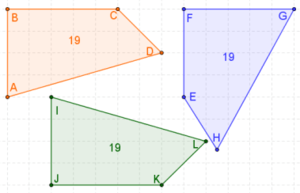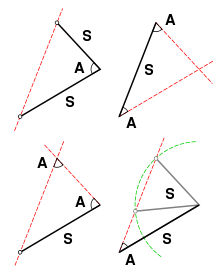# Congruence (geometry)An example of congruence. The two triangles on the left are congruent, while the third is similar to them. The last triangle is neither similar nor congruent to any of the others. Note that congruence permits alteration of some properties, such as location and orientation, but leaves others unchanged, like distance and angles. The unchanged properties are called invariants.

In geometry, two figures or objects are congruent if they have the same shape and size, or if one has the same shape and size as the mirror image of the other.

More formally, two sets of points are called congruent if, and only if, one can be transformed into the other by an isometry, i.e., a combination of rigid motions, namely a translation, a rotation, and a reflection. This means that either object can be repositioned and reflected (but not resized) so as to coincide precisely with the other object. So two distinct plane figures on a piece of paper are congruent if we can cut them out and then match them up completely. Turning the paper over is permitted.

In elementary geometry the word congruent is often used as follows. The word equal is often used in place of congruent for these objects.

• Two line segments are congruent if they have the same length.
• Two angles are congruent if they have the same measure.
• Two circles are congruent if they have the same diameter.

In this sense, two plane figures are congruent implies that their corresponding characteristics are "congruent" or "equal" including not just their corresponding sides and angles, but also their corresponding diagonals, perimeters and areas.

The related concept of similarity applies if the objects differ in size but not in shape.

## Determining congruence of polygonsThe orange and green quadrilaterals are congruent; the blue is not congruent to them. All three have the same perimeter and area. (The ordering of the sides of the blue quadrilateral is "mixed" which results in two of the interior angles and one of the diagonals not being congruent.)

For two polygons to be congruent, they must have an equal number of sides (and hence an equal numberthe same numberof vertices). Two polygons with n sides are congruent if and only if they each have numerically identical sequences (even if clockwise for one polygon and counterclockwise for the other) side-angle-side-angle-... for n sides and n angles.

Congruence of polygons can be established graphically as follows:

• First, match and label the corresponding vertices of the two figures.
• Second, draw a vector from one of the vertices of the one of the figures to the corresponding vertex of the other figure. Translate the first figure by this vector so that these two vertices match.
• Third, rotate the translated figure about the matched vertex until one pair of corresponding sides matches.

If at any time the step cannot be completed, the polygons are not congruent.

## Congruence of triangles

Two triangles are congruent if their corresponding sides are equal in length, in which case their corresponding angles are equal in measure.

If triangle ABC is congruent to triangle DEF, the relationship can be written mathematically as:In many cases it is sufficient to establish the equality of three corresponding parts and use one of the following results to deduce the congruence of the two triangles.The shape of a triangle is determined up to congruence by specifying two sides and the angle between them (SAS), two angles and the side between them (ASA) or two angles and a corresponding adjacent side (AAS). Specifying two sides and an adjacent angle (SSA), however, can yield two distinct possible triangles.

### Determining congruence

Sufficient evidence for congruence between two triangles in Euclidean space can be shown through the following comparisons:

• SAS (Side-Angle-Side): If two pairs of sides of two triangles are equal in length, and the included angles are equal in measurement, then the triangles are congruent.
• SSS (Side-Side-Side): If three pairs of sides of two triangles are equal in length, then the triangles are congruent.
• ASA (Angle-Side-Angle): If two pairs of angles of two triangles are equal in measurement, and the included sides are equal in length, then the triangles are congruent.
The ASA Postulate was contributed by Thales of Miletus (Greek). In most systems of axioms, the three criteriaSAS, SSS and ASAare established as theorems. In the School Mathematics Study Group system SAS is taken as one (#15) of 22 postulates.
• AAS (Angle-Angle-Side): If two pairs of angles of two triangles are equal in measurement, and a pair of corresponding non-included sides are equal in length, then the triangles are congruent. (In British usage, ASA and AAS are usually combined into a single condition AAcorrS - any two angles and a corresponding side.) For American usage, AAS is equivalent to an ASA condition, by the fact that if any two angles are given, so is the third angle, since their sum should be 180°.
• RHS (Right-angle-Hypotenuse-Side), also known as HL (Hypotenuse-Leg): If two right-angled triangles have their hypotenuses equal in length, and a pair of shorter sides are equal in length, then the triangles are congruent.

#### Side-side-angle

The SSA condition (Side-Side-Angle) which specifies two sides and a non-included angle (also known as ASS, or Angle-Side-Side) does not by itself prove congruence. In order to show congruence, additional information is required such as the measure of the corresponding angles and in some cases the lengths of the two pairs of corresponding sides. There are a few possible cases:

If two triangles satisfy the SSA condition and the length of the side opposite the angle is greater than or equal to the length of the adjacent side (SsA, or long side-short side-angle), then the two triangles are congruent. The opposite side is sometimes longer when the corresponding angles are acute, but it is always longer when the corresponding angles are right or obtuse. Where the angle is a right angle, also known as the Hypotenuse-Leg (HL) postulate or the Right-angle-Hypotenuse-Side (RHS) condition, the third side can be calculated using the Pythagorean Theorem thus allowing the SSS postulate to be applied.

If two triangles satisfy the SSA condition and the corresponding angles are acute and the length of the side opposite the angle is equal to the length of the adjacent side multiplied by the sine of the angle, then the two triangles are congruent.

If two triangles satisfy the SSA condition and the corresponding angles are acute and the length of the side opposite the angle is greater than the length of the adjacent side multiplied by the sine of the angle (but less than the length of the adjacent side), then the two triangles cannot be shown to be congruent. This is the ambiguous case and two different triangles can be formed from the given information, but further information distinguishing them can lead to a proof of congruence.

#### Angle-angle-angle

In Euclidean geometry, AAA (Angle-Angle-Angle) (or just AA, since in Euclidean geometry the angles of a triangle add up to 180°) does not provide information regarding the size of the two triangles and hence proves only similarity and not congruence in Euclidean space.

However, in spherical geometry and hyperbolic geometry (where the sum of the angles of a triangle varies with size) AAA is sufficient for congruence on a given curvature of surface.

## Definition of congruence in analytic geometry

In a Euclidean system, congruence is fundamental; it is the counterpart of equality for numbers. In analytic geometry, congruence may be defined intuitively thus: two mappings of figures onto one Cartesian coordinate system are congruent if and only if, for any two points in the first mapping, the Euclidean distance between them is equal to the Euclidean distance between the corresponding points in the second mapping.

A more formal definition states that two subsets A and B of Euclidean space Rn are called congruent if there exists an isometry f : RnRn (an element of the Euclidean group E(n)) with f(A) = B. Congruence is an equivalence relation.

## Congruent conic sections

Two conic sections are congruent if their eccentricities and one other distinct parameter characterizing them are equal. Their eccentricities establish their shapes, equality of which is sufficient to establish similarity, and the second parameter then establishes size. Since two circles, parabolas, or rectangular hyperbolas always have the same eccentricity (specifically 0 in the case of circles, 1 in the case of parabolas, andin the case of rectangular hyperbolas), two circles, parabolas, or rectangular hyperbolas need to have only one other common parameter value, establishing their size, for them to be congruent.

## Congruent polyhedra

For two polyhedra with the same number E of edges, the same number of faces, and the same number of sides on corresponding faces, there exists a set of at most E measurements that can establish whether or not the polyhedra are congruent. For cubes, which have 12 edges, only 9 measurements are necessary.

## Congruent triangles on a sphere

As with plane triangles, on a sphere two triangles sharing the same sequence of angle-side-angle (ASA) are necessarily congruent (that is, they have three identical sides and three identical angles). This can be seen as follows: One can situate one of the vertices with a given angle at the south pole and run the side with given length up the prime meridian. Knowing both angles at either end of the segment of fixed length ensures that the other two sides emanate with a uniquely determined trajectory, and thus will meet each other at a uniquely determined point; thus ASA is valid.

The congruence theorems side-angle-side (SAS) and side-side-side (SSS) also hold on a sphere; in addition, if two spherical triangles have an identical angle-angle-angle (AAA) sequence, they are congruent (unlike for plane triangles).

The plane-triangle congruence theorem angle-angle-side (AAS) does not hold for spherical triangles. As in plane geometry, side-side-angle (SSA) does not imply congruence.

1. Clapham, C.; Nicholson, J. (2009). "Oxford Concise Dictionary of Mathematics, Congruent Figures" (PDF). Addison-Wesley. p. 167. Retrieved September 2013. Check date values in: |access-date= (help)
2. "Congruence". Math Open Reference. 2009. Retrieved September 2013. Check date values in: |access-date= (help)Wikimedia Commons has media related to Congruence.